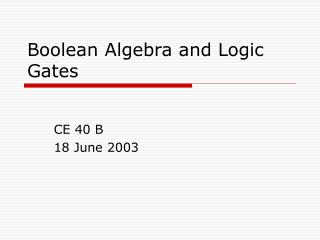DownloadDownload PresentationBoolean Algebra and Logic Gates

# Boolean Algebra and Logic Gates

Download Presentation## Boolean Algebra and Logic Gates

- - - - - - - - - - - - - - - - - - - - - - - - - - - E N D - - - - - - - - - - - - - - - - - - - - - - - - - - -
##### Presentation Transcript

1. Boolean Algebra and Logic Gates CE 40 B 18 June 2003

2. Basic Definitions • Common Postulates • Closure • Associative Law • Commutative Law • Identity Element • Inverse • Distributive Law • Field • set of elements with two binary operators

3. Axiomatic Definition of Boolean Algebra • George Boole • Two binary operators (+ and ·) • Huntington Postulates • Closure, Identity, Commutative, Distributive, Complement • Show that Huntington Postulates are valid for B = {0,1}

4. Basic Theorems and Properties of Boolean Algebra • Duality • Expression remains valid even after operators and identity elements are interchanged • Some theorems • x + xy = x • (x + y)’ = x’y’

5. Boolean Functions • Logical relationship between binary variables • Can be represented by a truth table • Can be transformed into a logic diagram • Example: f = x + y’z

6. Example – f = x + y’z

7. Canonical and Standard Forms • Maxterms and Minterms • Canonical Forms • Sum of Minterms • Product of Maxterms • Standard Forms • Sum of products • Product of sums

8. Other Logic Operations • Formed from combination AND, OR, and NOT. • 16 possible functions for 2 binary variables

9. Digital Logic Gates

10. Integrated Circuits • IC • Silicon chip containing electronic components for constructing digital gates • Different Levels of Integration • Circuit complexity • Number of logic gates in one package • SSI, MSI, LSI, VLSI

11. Integrated Circuits • Digital Logic Families • Circuit technology – how the logic gates are constructed • TTL, ECL, MOS, CMOS • Characteristics • Fan-out • Fan-in • Power dissipation • Propagation delay • Noise margin

12. Integrated Circuits • Computer-Aided Design • Complex designs require computers • Electronic Design Automation (EDA) • Hardware Description Language (HDL) • Verilog, VHDL